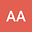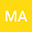Asymptotic analysis for a nonlinear viscoelastic problem with innite history under a wider class of relaxation functions
••In this paper, we consider a nonlinear viscoelastic problem with infinite history and a nonlinear feedback localized on the domain and a relaxation function satisfying $$g^{\prime}(t) \le -\xi(t)G(g(t)).$$ We establish explicit and general decay rate results, using the multiplier method and some properties of the convex functions. Our results are obtained without imposing any restrictive growth assumption on the damping term and without imposing any assumption on the boundedness of initial data used in many earlier papers in the literature.